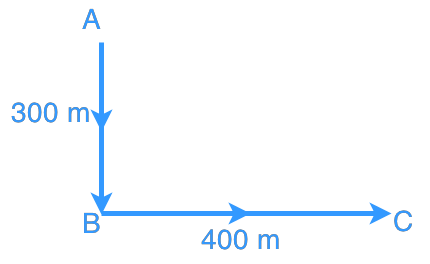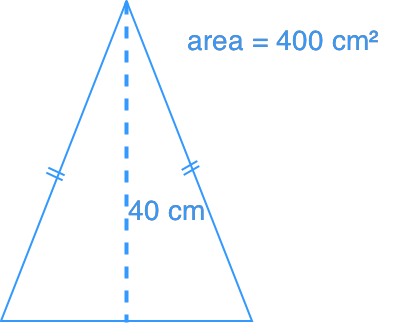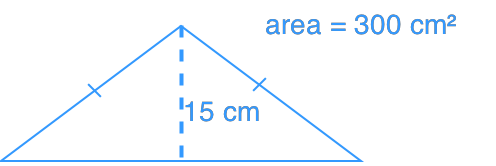# Applications of pythagorean theorem#### Everything You Need in One Place

Homework problems? Exam preparation? Trying to grasp a concept or just brushing up the basics? Our extensive help & practice library have got you covered.#### Learn and Practice With Ease

Our proven video lessons ease you through problems quickly, and you get tonnes of friendly practice on questions that trip students up on tests and finals.#### Instant and Unlimited Help

Our personalized learning platform enables you to instantly find the exact walkthrough to your specific type of question. Activate unlimited help now!##### Intros
###### Lessons
1. i) What is Pythagorean Theorem?

ii) How to use Pythagorean Theorem to find sides of a triangle?

2. What are Pythagorean Triples?
##### Examples
###### Lessons
1. An aeroplane flew from point A to point B, and then to Point C. How far is the plane from point A? Refer to the diagram below.1. A 2.5 m long ladder is put against a wall. If the base of the ladder is 1.25 m away from the wall, what is the length from the ground to the point where the top of ladder touches the wall?
1. Which triangle has a longer perimeter?
Triangle ATriangle B##### Practice
###### Topic Notes
We will need to apply Pythagorean Theorem often in our daily life. In this lesson, we will focus on tackling some Pythagorean Theorem word problems.